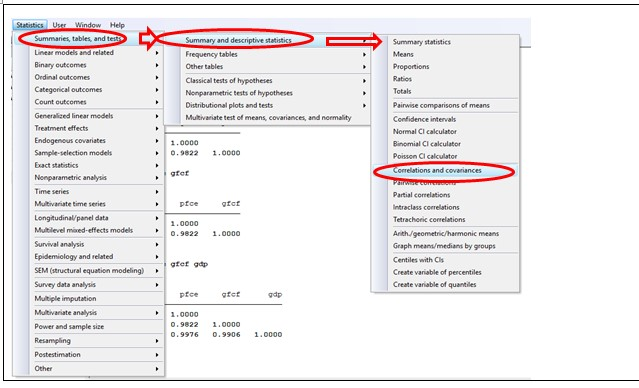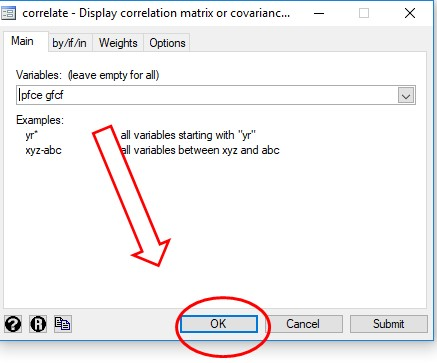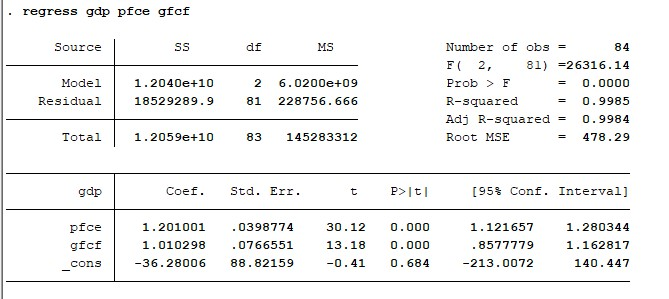# How to test time series multicollinearity in STATA?

After performing autocorrelation tests in STATA in the previous article, this article will explain the steps for detecting multicollinearity in time series. The problem of multicollinearity arises when one explanatory variable in a multiple regression model highly correlates with one or more than one of other explanatory variables. It is a problem because it underestimates the statistical significance of an explanatory variable (Allen, 1997). A high correlation between independent variables will result in a large standard error. This will make the corresponding regression coefficients unstable and also statistically less significant.

## How to detect multicollinearity?

There are three methods to detect:

### 1. Checking the correlation between all explanatory variables

Check correlation between all the explanatory variables. If there is a high correlation between the independent variables, then there exists multicollinearity.

In order to do this, follow the below steps as shown in the figure below.

• Go to ‘Statistics’.
• Click on ‘Summaries, tables and tests’.
• Go to ‘Summary and descriptive statistics’.
• Click on ‘Correlations and covariances’.Figure 1: Procedure to detect multicollinearity

Alternatively, type the below STATA command:

`correlate (independent variables)`

This article uses the same dataset as the previous article (Testing for time series autocorrelation in STATA). Therefore in the dialogue box of correlate, enter the independent variables ‘pfce’ and ‘gfcf’.Figure 2: Selection of variables for detecting multicollinearity in STATA

Click on ‘OK’.  The following result will appear.Figure 3: Result of multicollinearity in STATA

The correlation value comes out to be 0.9822, which is very close to 1. Thus there is a high degree of correlation between variables PFC and GFC.

### 2. Inconsistency in significance values

The second method is when individual statistics values in the regression results come out to be insignificant but their joint statistics value is significant. It also indicates that there is multicollinearity which undermines the individual significance, as explained at the beginning of this article.

### 3. Using vif command

The third method is to use ‘vif’ command after obtaining the regression results. ‘vif’ is the variance inflation factor which is a measure of the amount of multicollinearity in a set of multiple regression variables. It is a good indicator in linear regression. The figure below shows the regression results.Figure 3: Regression results using vif command in STATA

Use the command in the prompt as follows:

`vif`

The below result will appear.Figure 4: Result of multicollinearity in STATA using vif command

Here the mean vif is 28.29, implying that correlation is very high. As a rule of thumb, vif values less than 10 indicates no multicollinearity between the variables. 1/vif is the tolerance, which indicates the degree of collinearity. Variables with tolerance value less than 0.1 are the linear combination of other explanatory variables, which turns out to be the case here for both PFC and GFC.

Since GFC and PFC are highly correlated with each other, there is a presence of multicollinearity in the model.

## Correction for multicollinearity in STATA

There is no specific command in STATA to correct the problem of multicollinearity. However, the following procedures help deal with the issue.

1. Remove highly correlating variables.
2. Linearly combine the independent variables, such as adding them together.
3. Perform an analysis for highly correlating variables, such as principal components analysis or partial least squares regression.
4. Transform functional form of the linear regression such as converting functional form in log-log, lin-log, log-lin among others.

This article completes the diagnostic tests for time series analysis, thus concluding the section of time series on this STATA module.

### Rashmi Sajwan

Research Analyst at Project Guru
Rashmi has completed her bachelors in Economic (hons.) from Delhi University and Masters in economics from Guru Gobind Singh Indrapastha University. She has good understanding of statistical softwares like STATA, SPSS and E-views. She worked as a Research Intern at CIMMYT international maize and wheat improvement centre. She has an analytical mind and can spend her whole day on data analysis. Being a poetry lover, she likes to write and read poems. In her spare time, she loves to do Dance.

#### Latest posts by Rashmi Sajwan (see all)We are looking for candidates who have completed their master's degree or Ph.D. Click here to know more about our vacancies.